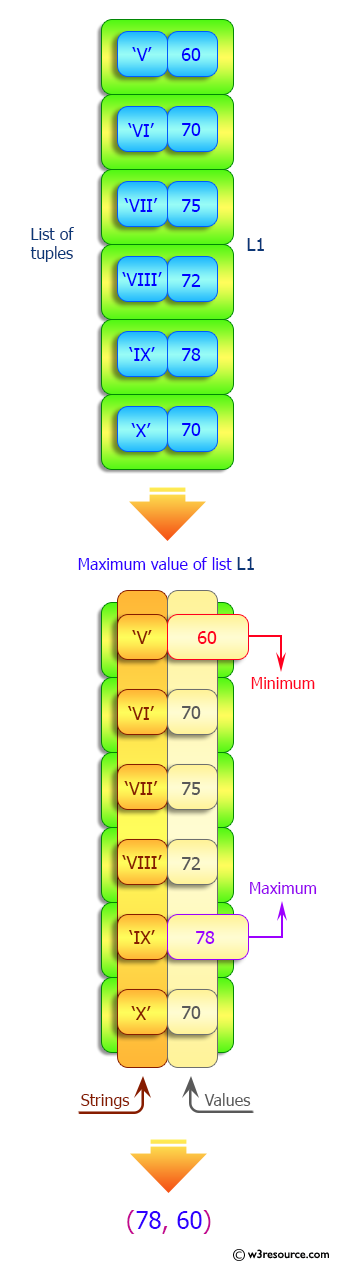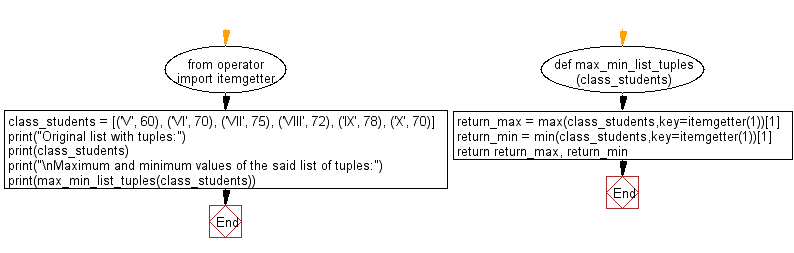﻿ Python: Find the maximum and minimum values in a given list of tuples - w3resource# Python: Find the maximum and minimum values in a given list of tuples

## Python List: Exercise - 158 with Solution

Write a Python program to find the maximum and minimum values in a given list of tuples.

Sample Solution:

Python Code:

``````from operator import itemgetter

def max_min_list_tuples(class_students):
return_max = max(class_students,key=itemgetter(1))
return_min = min(class_students,key=itemgetter(1))
return return_max, return_min

class_students = [('V', 60), ('VI', 70), ('VII', 75), ('VIII', 72), ('IX', 78), ('X', 70)]
print("Original list with tuples:")
print(class_students)
print("\nMaximum and minimum values of the said list of tuples:")
print(max_min_list_tuples(class_students))
```
```

Sample Output:

```Original list with tuples:
[('V', 60), ('VI', 70), ('VII', 75), ('VIII', 72), ('IX', 78), ('X', 70)]

Maximum and minimum values of the said list of tuples:
(78, 60)
```

Pictorial Presentation:Flowchart:## Visualize Python code execution:

The following tool visualize what the computer is doing step-by-step as it executes the said program:

Python Code Editor:

Have another way to solve this solution? Contribute your code (and comments) through Disqus.

What is the difficulty level of this exercise?

Test your Python skills with w3resource's quiz

﻿

## Python: Tips of the Day

Floor Division:

When we speak of division we normally mean (/) float division operator, this will give a precise result in float format with decimals.

For a rounded integer result there is (//) floor division operator in Python. Floor division will only give integer results that are round numbers.

```print(1000 // 300)
print(1000 / 300)```

Output:

```3
3.3333333333333335```